# Grade 12 Compound and Simple Interest .

Introduction

Topic

Simple interest and description of formula ,Compound interest and description of formula

INTEREST

• Interest is the fixed amount paid on borrowed money.
• The sum lent is called the Principal.
• The sum of the principal and interest is called the Amount.
• Interest is of two kinds:
1. Simple interest  2. Compound interest

1.Simple interests :  In the Real life situation when money is deposited in a bank or building society account, it commonly attracts interest; in a similar way, a borrower must normally pay interest on money borrowed. The rate of interest is usually (but not always)percent per year , half yearly and quarterly  .

2. Compound interest: Simple interest is very rarely used in real life: almost all banks and other financial institutions use compound interest. This is when interest is added (or compounded) to the principal sum so that interest is paid on the whole amount. Under this method, if the interest for the first year is left in the account, the interest for the second year is calculated on the whole amount so far accumulated . The rate of interest is usually (but not always)percent per year , Monthly ,half yearly and quarterly  .

•  Observe the diagrams below and compare the diffference .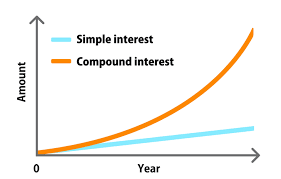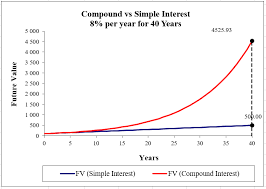• 07 September 2021.
• total marks of 12.

•  Mr .Sondlo  wants to buy a television on a hire purchase agreement. The cash price of the television is R5 600. He  is required to pay a deposit of 15% and pay the remaining loan amount off over 24 months at an interest rate of 14% p.a.

1.Calculate the total mount that Mr November is going to pay at the end of 24  months.(4)

2.  Calculate the amount of Deposit that he is going to pay upfront (2)

• An amount of R3 500 is invested in a savings account which pays a compound interest rate of 7,5% p.a.

1. Calculate the balance accumulated by the end of 2 years.(4)

2.What is going to be a yearly interest on the investment.(2)

in order to validate the errors on your calculation look at the below example .

.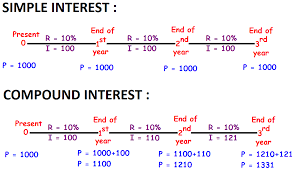(12) total marks.

Process

Interest is defined as the cost of borrowing money, as in the case of interest charged on a loan balance. Conversely, interest can also be the rate paid for money on deposit, as in the case of a certificate of deposit. Interest can be calculated in two ways: simple interest or compound interest.

• Simple interest is calculated on the principal, or original, amount of a loan.
• Compound interest is calculated on the principal amount and the accumulated interest of previous periods, and thus can be regarded as interest on interest.

Formulae :

• Simple interest = (Principal×Time×Rate)/100
i.e. S.I. = (P×R×T)/100

Principal(P) , Rate(R), Time(T), Simple Interests (S.I).

Formulae :

• Compound Interest A=P(1+i)n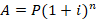Example : Find the compound interest on 25625 for 12 months at 16% per annum, compound quarterly.

A=P(1+(r/4)/100)^(4×n)=P(1+r/400)^4n

Expand your understanding even by watching this two videos below .

Evaluation

1.

1 .A=P(1+in)

=R4 760,00(1+0,14×2)

=R6 092,80

Total amount=A+deposit

amount=R6 092,80+840

2.Deposit =15/100*R5600

=R840.

• 1. PinA=?

A=3 500

=0,075

=2=P(1+i)n

=3 500(1+0,075)2

=R4 044,69

• 2.Interest = 7.5/100*R350  =R262.55

•

Conclusion

Students after you have read and wrote the activity of compound and simple interest please watch this videos on your spare time as we are going to learn the new topic in the class also look at the example provided .

Conversions and Time calculate .

• Examples .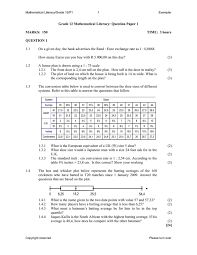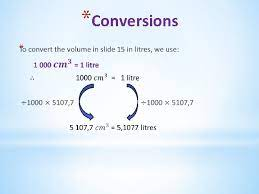Credits

References of the information.

Reference for the information .

• Mathematical Literacy spot on grade 12 learners book .